### REIFY Statement

`REIFY` reify_constraint-1 <…reify_constraint-n> ;

where reify_constraint is specified in the following form:

variable : constraint

The REIFY statement associates a binary variable with a constraint. The value of the binary variable is 1 or 0 depending on whether the constraint is satisfied or not, respectively. The constraint is said to be reified, and the binary variable is referred to as the control variable. Currently, the only type of constraint that can be reified is the linear constraint, which should have the same form as linear_constraint defined in the LINCON statement. As with the other variables, the control variable must also be defined in a VARIABLE statement or in the CONDATA= data set.

The REIFY statement provides a convenient mechanism for expressing logical constraints, such as disjunctive and implicative constraints. For example, the disjunctive constraint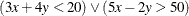can be expressed with the following statements:

```var x y p q;
reify p: (3 * x + 4 * y < 20) q: (5 * x - 2 * y > 50);
lincon p + q >= 1;
```

The binary variables
and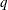reify the linear constraints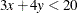and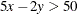respectively. The following linear constraint enforces the desired disjunction: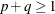The implication constraint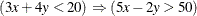can be enforced with the linear constraint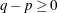The REIFY constraint can also be used to express a constraint that involves the absolute value of a variable. For example, the constraint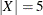can be expressed with the following statements:

```var x p q;
reify p: (x = 5) q: (x = -5);
lincon p + q = 1;
```